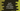# Different return types in swift functions### Swift function without return values :

We can write functions without any return values . That means, if you call the function, it will return no value and the program will continue running from the next line. Example :

``````func sayHello() {
print ("Hello")
}
sayHello()``````

It will print ’Hello’ as output. So, mainly we called one function and it printed one line. That’s it.

### Swift function with a return value :

For the above example, instead of printing the string ’Hello’ , we can ask it to return the string to the caller function. We will store it in a variable and print out the result.

``````func returnHello() -> String {
return "Hello"
}

let returnString = returnHello()
print (returnString)``````

The output of the above program is same as the previous one i.e. ”Hello”. ’returnHello’ function returns ’Hello’ String . If a function returns something, we write the name as ‘func functionName() -> returnValue {}’ . Here, ’returnHello’ function returns a string, so we have ’-> String’ after the function name. So, on calling this function, it returns one string. We are using one constant ’returnString’ to hold that return value. On last statement, we are just print the value of ’returnString’ i.e. the return value of ’returnHello’ function.

### Returning multiple values from function in swift :

In swift, we can even return multiple values from a function. We can return the values as tuple to the caller. Following example will clarify your doubts :

``````func findTopThree(array : [Int]) ->(first : Int, second : Int, third : Int){
var currentFirst = -1
var currentSecond = -1
var currentThird = -1

for value in 0...array.count-1{
if (array[value] >= currentFirst){
currentThird = currentSecond
currentSecond = currentFirst
currentFirst = array[value]
}else if (array[value] > currentSecond){
currentThird = currentSecond
currentSecond = array[value]
}else if(array[value] > currentThird){
currentThird = array[value]
}
}

return (currentFirst,currentSecond,currentThird)
}

let marks = [35, 46, 34, 29, 59, 70, 82, 89, 45,80,95, 87,23, 49,91]

let result = findTopThree(array:marks)
print ("Top mark : \(result.first)")
print ("Second mark : \(result.second)")
print ("Third mark : \(result.third)")``````

Output :

``````Top mark : 95
Second mark : 91
Third mark : 89``````

In this example, we are finding out the top three marks from an array of marks. The return value of the function is defined as ’->(first : Int, second : Int, third : Int)’ i.e. it will return three integers in a tuple with name ’first’, ’second’ and ’third’. We created one constant ’result’ to hold these values. Since, the values of a tuple are named same as the return type defined in the function, we can access each one of them using a dot ’.’ . The last three print lines are used to print out the ’first’ , ’second’ and ’third’ values of that tuple.

### Returning Optional Tuple from a Swift function :

We can also return optional tuple values from a function in swift. Optional means it may or mayn’t have any return values. For example, check the below program :

``````func findFirstTwoNegativeNumbers(array : [Int]) -> (first : Int, second : Int)? {
var firstNumber = 0
var secondNumber = 0

for value in 0...array.count - 1 {
if(array[value] < 0){
if firstNumber == 0 {
firstNumber = array[value]
}else if secondNumber == 0{
secondNumber = array[value]
return (firstNumber,secondNumber)
}
}
}
return nil
}

let numbers = [ 1,2,3,4,5]
print("First two negative numbers \(findFirstTwoNegativeNumbers(array : numbers))")

let numbers2 = [ 1,2,3,-1,4,5,-4,0,9,-5]
print("First two negative numbers \(findFirstTwoNegativeNumbers(array : numbers2))")``````

Output :

``````First two negative numbers nil
First two negative numbers Optional((first: -1, second: -4))``````

This function is used to find out the first two negative numbers in an array. Here , the return type is ‘(first : Int, second : Int)? ’ _i.e. it may or mayn’t return results. If it will find _two negative numbers in the input array, it will return these as a tuple. If one or no negative number is found, it will return ‘nil’. For the first example, since all the numbers are positive, it returns ‘nil’.

That’s all for today. I will write more articles on function in Swift. Keep visiting codevscolor. Happy Coding :)